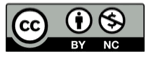# Python all和any函数对空列表的返回值

2014-10-23Python有一对内置的函数: all()和any()，他们两个都接收可遍历的对象（也就是列表list、元组tuple之类的），all检查所有元素是否全都为真，any检查至少有一个元素为真。本文简单分析它们的用法。

all(iterable)

Return True if all elements of the iterable are true (or if the iterable is empty).

any(iterable)

Return True if any element of the iterable is true. If the iterable is empty, return False.

``````any(a, b, c, ..., z)
= a + any(b, c, ..., z)
= a + b + any(c, ..., z)
= ...
= a + b + c + ... + z + any([])
``````

``````all(a, b, c, ..., z)
= a * any(b, c, ..., z)
= a * b * any(c, ..., z)
= ...
= a * b * c * ... * z * all([])
``````

all函数的这种特性产生了一个后果：当检查一个序列都是真值时，必须先检查非空，不然可能会漏过空序列：

``````if matched and all(matched):
# do something...
``````

## 精彩评论